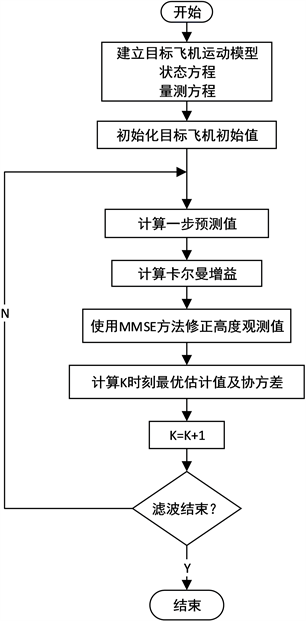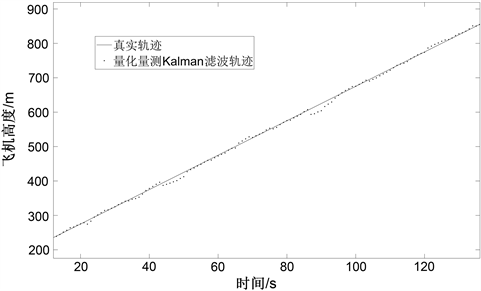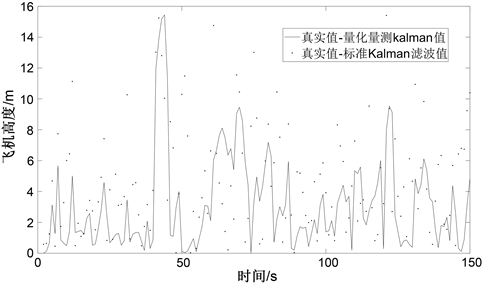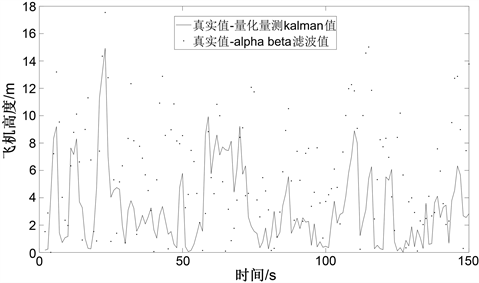# 机载防撞系统ACAS X高度跟踪器目标跟踪算法Target Tracking Algorithm of Airborne Collision Avoidance System ACAS X Height Tracker

DOI: 10.12677/MOS.2021.104092, PDF, HTML, XML, 下载: 21  浏览: 62  科研立项经费支持

Abstract: Whether the height tracker can effectively work on the vertical plane of the new generation of air-borne collision avoidance system (ACAS X) is the key to the design. In traditional research, inaccurate height rate estimation is a drawback of nonlinear trackers, and linear trackers have deteriorated accuracy due to the “coarse quantization” reported in the report. In order for the tracker to process the target aircraft state more stably, the research uses quantitative measurement state estimation algorithm, which describes the aircraft state uncertainty as a probability distribution, quantizes it as Gaussian noise and uses Sheppard correction, and estimates the state of the aircraft on the vertical plane through the Kalman filter algorithm. Experimental results show that the algorithm is superior to traditional designs in high tracking accuracy, can effectively make anti-collision decisions, and provide higher reliability for ACAS X.

1. 引言

2. α-β跟踪器滤波原理

α-β滤波器是针对匀速运动目标的一种常增益滤波器，其形式和Kalman滤波器相似。经过一步预测得到的预测值，其值与测量值之间存在的残差，记为 ${\stackrel{^}{r}}_{k}$。当目标飞机数据传入高度跟踪器时，采用选定的α和β常数，使用残差的α倍校正飞机的高度估计，使用β乘以残差来校正飞机的高度率估计：

${\stackrel{^}{z}}_{k}={\stackrel{^}{z}}_{k|k-1}+\alpha {\stackrel{^}{r}}_{k}$ (1)

${\stackrel{^}{\stackrel{˙}{z}}}_{k}={\stackrel{^}{\stackrel{˙}{z}}}_{k|k-1}+\left(\beta /T\right){\stackrel{^}{r}}_{k}$ (2)

3. 非线性高度跟踪器滤波原理

$z\left(t\right)=Nq+{\epsilon }_{0}q+\stackrel{˙}{z}t$ (3)

$T=\frac{q}{|\stackrel{˙}{z}|}$ (4)

$\stackrel{^}{\stackrel{˙}{z}}={d}_{t}\frac{q}{\stackrel{^}{T}}$ (5)

4. ACAS X高度跟踪器

TCAS系统的跟踪器旨在产生垂直速率的单点估计，而ACAS X是在TCAS II的基础上升级的新一代机载防撞系统，将飞机状态的步确定性考虑在内，这样可以改善TCAS设计的局限性，优化成本函数和决策逻辑，大大提高防撞性能和降低报警率 。ACAS X的跟踪器沿用了TCAS跟踪器的设计思路，将复杂的三维空间目标跟踪问题分解为水平和垂直两个维度，分别设计了水平跟踪器和垂直跟踪器。垂直跟踪器也称为高度跟踪器，用于对目标飞机的高度和高度率进行状态估计。

4.1. 高度跟踪器算法流程

Step 1：建立目标飞机的运动模型，得到目标飞机的状态方程和量测方程；

Step 2：对目标飞机的初始状态和误差的协方差进行初始化；

Step 3：使用k − 1时刻的估计值，对k时刻目标的状态进行一步预测，并计算一步预测误差的协方差；

Step 4：由给出的系统模型中的变量及一步预测误差协方差，计算Kalman增益；

Step 5：引入观测值、预测值以及量化区间，使用MMSE状态估计算法；

Step 6：更新k时刻目标的状态估计，并且更新k时刻状态误差的协方差。Figure 1. ACAS X height tracker data processing flow

4.2. 高度的量化量测Kalman状态估计

$d=\underset{i=1}{\overset{L}{\sum }}{\text{e}}^{-\frac{{\left(g\left(i\right)-\mu \right)}^{2}}{2{\sigma }^{2}}}$ (6)

Step 1：对高度的观测值z进行处理。将k时刻获得的高度观测值z进行分割，量化量测值z对应的取值区间为： $\left[z-\frac{q}{2},z+\frac{q}{2}\right]$，把量化量测值进行分割就是将区间分为L个高度分区，可求得每个分区的中点值为：

$g\left(i\right)=z+\frac{1}{L}\left[\left(2i-1\right)-L\right]\frac{q}{2}$ (7)

Step 2：假设目标飞机的高度观测值落在每个分区的概率符合高斯分布，利用高斯分布的概率公式，求出落在每个分区中间点g(i)的概率密度.在计算高斯分布的概率密度时，采用量测的一步预测值及其预测误差的协方差，作为高度观测值的均值和方差。

$p\left(i\right)=\frac{1}{d}{\text{e}}^{-\frac{{\left(g\left(i\right)-\mu \right)}^{2}}{2{\sigma }^{2}}}$ (8)

Step 3：利用最小均方误差公式，以及离散型随机变量的均值和方差公式，计算每个分区的中心点g(i)偏离高度估计值 $\stackrel{^}{z}$ 的均值和方差，从而得到高度观测值的均值以及高度观测误差的方差。

${{z}^{\prime }}_{k}=\underset{i=1}{\overset{L}{\sum }}p\left(i\right)\left[g\left(i\right)-{\stackrel{^}{z}}_{k|k-1}\right]$ (9)

${{R}^{\prime }}_{k}=E\left[{\left(g\left(i\right)-{\stackrel{^}{z}}_{k|k-1}\right)}^{2}\right]-{\left(E\left[g\left(i\right)-{\stackrel{^}{z}}_{k|k-1}\right]\right)}^{2}$ (10)

5. 仿真结果分析

${x}_{k}=F{x}_{k-1}+G{w}_{k-1}$ (11)

${z}_{k}=H{x}_{k}+{v}_{k}$ (12)

1) 当高度报告为25 ft时，三种滤波效果误差近似。为了仿真效果更明显，按民航飞机飞行，设定飞机初始化高度为180 m开始匀速上升飞行，高度率为200 m/s，量化步长q = 100 ft，按照4.1小节高度跟踪器的处理流程，得到经量化量测Kalman滤波处理后的高度轨迹如图2所示。Figure 2. Quantitative measurement of Kalman filter simulation effect diagram

2) C模式下目标飞机的跟踪性能较差，且估计的高度滤波存在振荡行为，尤其在标准Kalman滤波中该行为影响较大。针对这一问题，为了验证本文4.1小节所述方法的有效性，使用同一条件仿真，并将结果与标准Kalman滤波的结果对比。图3为量化量测Kalman滤波与标准Kalman滤波对高度估计误差的对比。Figure 3. Quantized measurement Kalman filter and the standard Kalman filter error comparison diagram

3) TCAS系统中α、β在仿真中分别设定值为0.27和0.4，此值满足系统稳定性要求，且是在相同条件下滤波效果最好的取值。为了验证本文4.1小节所述方法的有效性，使用同一条件仿真，并将结果与TCAS系统中α-β滤波器的结果对比。图4为量化量测Kalman滤波与α-β滤波对高度估计的误差的对比。Figure 4. Quantified measurement of Kalman filter and α-β filter error comparison diagram

6. 结束语

NOTES

*通讯作者。

  (1980) Description of BCAS Altitude Tracker (Version VTRA 66). No. 42C-2020. MIT Lincoln Laboratory, Lexington, MA.  林云松, 郝中豪, 彭良福, 等. 机载防撞系统非线性高度跟踪器的设计与实现[J]. 电讯技术, 2015, 55(5): 552-559.  Asmar, D.M., Kochenderfer, M.J., et al. (2013) Vertical State Estimation for Aircraft Collision Avoidance with Quantized Measurements. Journal of Guidance, Control, and Dynamics, 36, 1801-1806. https://doi.org/10.2514/1.58938  Gardner, R.W., Genin, D. and Mcdowell, R. (2016) Probabilistic Model Checking of the Next-Generation Airborne Collision Avoidance System. 2016 IEEE/AIAA 35th Digital Avionics Systems Conference (DASC), Sacramento, CA, 25-29 September 2016, 1-10. https://doi.org/10.1109/DASC.2016.7777963  黄鹤, 张会生, 许家栋, 黄莺, 闫学斌. 一种改进的α-β-γ滤波跟踪算法[J]. 西北工业大学学报, 2008(2): 146-151.  RTCA (2018) Minimum Operational Performance Standards for Airborne Collision Avoidance System X (ACAS X) (ACAS Xa and ACAS Xo) Volume II, DO-385.  Priess, S., Weitz, L.A., Haissig, C.M., et al. (2020) TCAS II and ACAS Xa Traffic and Resolution Advisories during Interval Management Paired Approach Operations. 2020 AIAA/IEEE 39th Digital Avionics Systems Conference (DASC), San Antonio, TX, 11-15 October 2020, 1-8. https://doi.org/10.1109/DASC50938.2020.9256521  李铜波. 综合化防撞系统混合监视的设计与实现[D]: [硕士学位论文]. 成都: 电子科技大学, 2020.  张鸿麟. 基于MDP的通用航空机载防撞逻辑的设计[D]: [硕士学位论文]. 成都: 电子科技大学, 2019.  杨秀玉. 基于5G移动通信的无人机与民用飞机防相撞技术研究[D]: [硕士学位论文]. 广汉: 中国民用航空飞行学院, 2019.  吴凡. 基于非线性滤波的机动目标跟踪算法研究[D]: [硕士学位论文]. 西安: 西安电子科技大学, 2010.